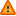FAQ/wmeans - CBU statistics WikiYou should trust both wikis because the password could be read by the particular administrators.

Clear message
location: FAQ / wmeans

# A note about unequal group sizes in ANOVA

Howell (1992) pp 410-417 and 542-549 illustrates and recommends the use of the (default) Type III sums of squares (termed Method I by Howell) for looking at sources of variation in an anova where the group sizes differ. This is provided the differences in group sizes are independent of the groups.

The computations for these may be done using either a least squares approach based upon formulating the anova in terms of a linear regression (to generate Type III sums of squarres) or by using the formulae associated with equal groups as given, for example, here but replacing the i-th group sample size (\$\$n_text{i}\$\$) with its harmonic mean, n-bar(i)

n-bar(i) = Harmonic mean of k means = k/[(1/n1) + ... (1/nk)]

Howell (1997) states (e.g. on p.582 of his fourth edition) that using harmonic means of sample sizes to compute sums of squares in anovas in cases where group sizes are unequal gives sums of squares for sources of variation which closely approximate the type III sums of squares.

Using harmonic means, or Type III sums of squares, in an anova gives an unweighted means analysis which is an equally weighted analysis of group means because group means are treated equally irrespective of the size of the group upon which they are based. An example is here.

Tabachnick and Fidell pp 217-218 also discuss sums of squares. They, like Howell, recommend Type III sums of squares for use for equally weighted groups and type II sums of squares when the sample sizes reflect the importance of the groups, e.g. when it is desired to consider the relative frequency of their occurrence in the general population (giving rise to a weighted means approach).

Harmonic means are also useful for averaging rates - see this example.

References

Howell DC (1992) Statistical Methods for Psychology. Third Edition. Duxbury Press:Belmont, CA. (Fourth Edition is as above but published in 1997)

Tabachnick BG and Fidell LS (2007) Using Multivariate Statistics. Fifth Edition. Pearson:Boston.